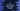# 3 different C# programs to print an inverted right-angled triangle## C# program to print inverted right-angled triangle:

Different ways in C# to print an inverted right-angled triangle in C#. We will learn how to use any character like star or any other character to print the triangle. We will also learn how to use numbers to print it. The user will enter the height of the triangle and the program will print the triangle on console.

### Algorithm:

The below algorithm is used to print the inverted right-angle triangle:

• Take the height of the triangle as an input from the user.
• Run one loop for height number of times.
• Run another inner loop to print the body of the triangle.
• For the first row, print height number of characters or numbers, for the second row, print height - 1 number of characters/numbers etc.
• Print one new line at the end of each iteration of the inner loop to move to the next line.

For example, if the height is 6, the triangle will look as like below:

``````* * * * * *
* * * * *
* * * *
* * *
* *
*``````

### Example 1: C# program to print an inverted right-angled triangle with a character:

Let’s write a program to print the triangle with any character:

``````namespace Program
{
class Program
{
static void Main(string[] args)
{
int Height;
char Char = '*';
Console.WriteLine("Enter the height of the triangle: ");

Console.WriteLine();

for (int i = 0; i < Height; i++)
{
for (int j = 0; j < Height - i; j++)
{
Console.Write(Char + " ");
}
Console.WriteLine();
}

}
}
}``````

Here,

• Height is the triangle height. The user entered the height and it is stored in the variable Height.
• Char is the character used to print the triangle.
• Two for loops are used to print the triangle. The outer for loop runs from i = 0 to i = Height - 1 i.e. it runs for Height number of times.
• The inner for loop runs for j = 0 to j = Height - i - 1 i.e. it runs for Height - i number of times.
• The character is printed inside the inner for loop. One new line is printed once the inner for loop ends. It moves to the next line.

If you run this program, it will print the output as like below:

``````Enter the height of the triangle:
7

* * * * * * *
* * * * * *
* * * * *
* * * *
* * *
* *
* ``````

You can also change the value of Char to use any other character to print the triangle.

``````Enter the height of the triangle:
7

\$ \$ \$ \$ \$ \$ \$
\$ \$ \$ \$ \$ \$
\$ \$ \$ \$ \$
\$ \$ \$ \$
\$ \$ \$
\$ \$
\$ ``````

### Example 2: C# program to print an inverted right-angled triangle of numbers:

We can also use numbers to print the triangle. Let’s change the above program to use numbers to print the triangle.

``````namespace Program
{
class Program
{
static void Main(string[] args)
{
int Height;
int Num;

Console.WriteLine("Enter the height of the triangle: ");

Console.WriteLine();

for (int i = 0; i < Height; i++)
{
Num = 1;
for (int j = 0; j < Height - i; j++)
{
Console.Write(Num + " ");
Num++;
}
Console.WriteLine();
}

}
}
}``````

Here, instead of a character, we are printing a number Num on the console. Its value is assigned to 1 before the inner for loop starts and on each iteration of the loop, it is incremented by 1. Its value is printed inside the for loop.

If you run this program, it will print output as like below:

``````Enter the height of the triangle:
7

1 2 3 4 5 6 7
1 2 3 4 5 6
1 2 3 4 5
1 2 3 4
1 2 3
1 2
1 ``````

We can also modify the program slightly to print the values in reverse.

``````namespace Program
{
class Program
{
static void Main(string[] args)
{
int Height;
int Num;

Console.WriteLine("Enter the height of the triangle: ");

Console.WriteLine();

for (int i = 0; i < Height; i++)
{
Num = Height;
for (int j = 0; j < Height - i; j++)
{
Console.Write(Num + " ");
Num--;
}
Console.WriteLine();
}

}
}
}``````

It will print:

``````Enter the height of the triangle:
7

7 6 5 4 3 2 1
7 6 5 4 3 2
7 6 5 4 3
7 6 5 4
7 6 5
7 6
7 ``````

### Example 3: C# program to print an inverted right-angled triangle using while loops:

Instead of for loops, we can also use while loops to print an inverted right-angled triangle.

``````namespace Program
{
class Program
{
static void Main(string[] args)
{
int Height, Num, i, j;

Console.WriteLine("Enter the height of the triangle: ");

Console.WriteLine();

i = 0;
while (i < Height)
{
Num = 1;
j = 0;
while (j < Height - i)
{
Console.Write(Num + " ");
Num++;
j++;
}
Console.WriteLine();
i++;
}

}
}
}``````

It works almost similar to the previous program. The while loop checks for a condition and if it is true, it runs its body. At the end of its iteration, the value of the variable is incremented by 1.

You will get similar result.